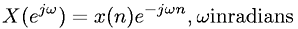Equations > Signal Processing > Fourier Transform Properties > Discrete-Time Fourier transform definition

### Discrete-Time Fourier transform definitionLatex Code:

MathML Code:

 $X\left({e}^{j\omega }\right)=x\left(n\right){e}^{-j\omega n},\omega \mathrm{in}\mathrm{radians}$

MathType 5.0: